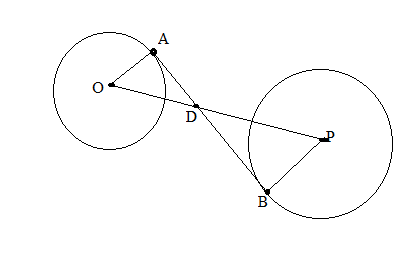Chapter 6.3, Problem 39EElementary Geometry For College St...

7th Edition
Alexander + 2 others
ISBN: 9781337614085

Solutions

Chapter
SectionElementary Geometry For College St...

7th Edition
Alexander + 2 others
ISBN: 9781337614085
Textbook Problem

The center of a circle of radius 2 in. is at a distance of 10 in. from the center of a circle of radius length 3 in. To the nearest tenth of an inch, what is the approximate length of a common internal tangent? Use the hint provided in Exercise 38.(HINT: use similar triangles to find OD and DP. Then apply the Pythagorean Theorem twice.)To determine

To find:

To find length of a common internal tangent.

Explanation

Given that, the center of a circle of radius 2 in. is at a distance of 10 in. from the center of a circle of radius length 3 in.

That is OA¯=2,PB¯=3andOP¯=10 then AB¯ is common internal tangent.

The diagrammatic representation is given below,

Since AB¯ is common internal tangent therefore, AB¯OA¯andAB¯PB¯

Using the vertical angle theorem to get the following,

If two triangles are similar, then the ration of any two corresponding segments (such as altitudes, medians, or angle bisectors) equals the ratio of any two corresponding sides.

OA¯PB¯=OD¯PD¯23=OD¯PD¯OD¯PD¯=233OD¯=2PD¯

We know that,

OP¯=OD¯+PD¯10=OD¯+PD¯PD¯=10OD¯. Since OP¯=10

Substitute this in the above equation to get the following,

Still sussing out bartleby?

Check out a sample textbook solution.

See a sample solution

The Solution to Your Study Problems

Bartleby provides explanations to thousands of textbook problems written by our experts, many with advanced degrees!

Get Started

In Exercises 3540, rationalize the numerator of each expression. 37. 133

Applied Calculus for the Managerial, Life, and Social Sciences: A Brief Approach

In Exercises 1728, use the logarithm identities to obtain the missing quantity.

Finite Mathematics and Applied Calculus (MindTap Course List)

Use the guidelines of Section 4.5 to sketch the curve. y=1x21(x2)2

Single Variable Calculus: Early Transcendentals, Volume I

Evaluate limx26x23x1.

Single Variable Calculus

I=ER,E=120,andR=12.findI.

Elementary Technical Mathematics

Find g(1)+g(2)+g(3)+....+g(50), given that g(x)=124x.

Finite Mathematics for the Managerial, Life, and Social Sciences

Arc Length Find the arc length of the graph of y=ln(cosx) from x=0tox=/3.

Calculus: Early Transcendental Functions (MindTap Course List)